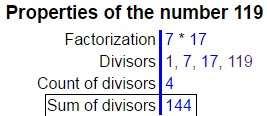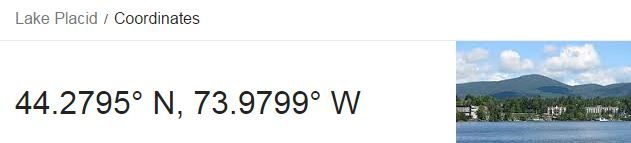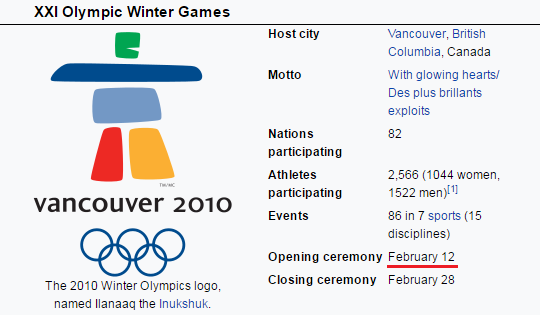## Cipher## May 7, 2017

### Bobsled driver Steven Holcomb dies - sacrifice?

Steven Holcomb was found dead on May 6th 2017, there's very little information about his death right now but it definitely looks like a planned sacrificeSteven Holcomb was born on April 14th a date that would be written 4/14 in US and 14/4 in most parts of the world
Fourteenth of April = 6+60+300+90+200+5+5+50+200+8 + 60+6 + 1+70+90+9+30 = 1190 (English Extended)
He died on May 6th 2017
May sixth = 13+1+25 + 19+9+24+20+8 = 119 (English Ordinal)Note that he was found dead in his room at the training center in Lake Placid = 12+1+11+5 + 16+12+1+3+9+4 = 74 (English Ordinal), died at 37 (74/2), Killing = 7444 connects to killing and 144 - Forty four = 6+15+18+20+25 + 6+15+21+18 = 144 (English Ordinal), notice how this connects with his birthday and the fact that he died in the sign of Taurus = 7+8+6+9+6+8 = 44 (Reverse Reduction)
He ended a 62 year drought in 2010 Winter Olympics and earned Gold Medal in four-man bobsled, "Gold Medal" = 2+3+6+5 + 5+4+5+8+6 = 44 (Reverse Reduction)Opening ceremony of the Olympic Winter games in 2010 came on a date with 44 numerology (2+12+20+10 = 44)
Bobsleigh = 2+50+2+90+20+5+9+7+8 = 193 (Jewish)
We have another connection to 44 in his place of birth (Park city = 70+1+90+20 + 3+9+200+700 = 1093 (English Extended), drop the zero and we are left with 44th prime number - 193)

Even the name of this website has 44 gematria.... http://www.teamholcomb.com/ - Team Holcomb = 2+5+1+4 + 8+6+3+3+6+4+2 = 44 (Full Reduction)

Honorable mention goes to CNN with their headline gematria as alwaysTeam USA bobsled driver Steven Holcomb dies = 153 (Full Reduction)
Steven Holcomb = 19+20+5+22+5+14 + 8+15+12+3+15+13+2 = 153 (English Ordinal)
Team USA bobsled driver Steven Holcomb dies = 216 (Reverse Reduction)
bobsled driver = 25+12+25+8+15+22+23 + 23+9+18+5+22+9 = 216 (Reverse Ordinal)
sixty two year drought = 8+18+3+7+2 + 7+4+12 + 2+22+26+9 + 23+9+12+6+20+19+7 = 216 (Reverse Ordinal)

Now here is the interesting part..Wikipedia says that he led the team to a gold medal victory on February 17th but all the news reports about the victory started coming in on the 27th and other Wikipedia links connecting to Bobsleigh competition talk about February 27th. I wonder if Wikipedia made honest mistake because 2/17 falls very interestingly with his time of death (measuring from 2/27/2010 to 5/6/2017 you find 7 years 2 months and 20 days)
February twenty seventh = 6+5+2+9+3+1+9+7 + 2+5+5+5+2+7 + 10+5+4+5+5+2+8 = 107 (Single Reduction)
Twenty two divided by seven = 2+5+5+5+2+7 + 2+5+6 + 4+9+4+9+4+5+4 + 2+7 + 1+5+4+5+5 = 107 (Full Reduction)We definitely find some 227 and connections to this competition
Olympic Winter Games = 15+12+25+13+16+9+3 + 23+9+14+20+5+18 + 7+1+13+5+19 = 227 (English Ordinal)
bobsled driver = 2+50+2+90+20+5+4 + 4+80+9+700+5+80 = 1051 (Jewish)If we measure from his last birthday to the time of his death we find 22 days but when we measure to his next birthday we find 343 days (octal of 227) or 344 days including the last date which is 11 months and 9 days..They chose a very special date to end this 62 year drought... and we have some 322's coming up as well
He died 22 days after his birthday - twenty two days = 200+500+5+50+200+700 + 200+500+60 + 4+1+700+100 = 3220 (English Extended)
Bobsleigh = 2+60+2+100+30+5+9+7+8 = 223 (English Extended)
sixty two years = 19+9+24+20+25 + 20+23+15 + 25+5+1+18+19 = 223 (English Ordinal)
Ending Bobsleigh drought after 62 years seems like we had some Skull and crossbones(223 Ordinal) involvement here, or maybe you could also call them "The synagogue of Satan" = 223 (English Ordinal)

From his birthday in 2009 until the end of the drought on 2/27/2010 is 320 daysNote that American team broke the drought on a date with 32 numerology (2+27+2+0+1+0 = 32) - America = 1+4+5+9+9+3+1 = 32 (Full Reduction)Bobsled = 2+6+2+10+3+5+4 = 32 (Single Reduction)Steven = 8+7+4+5+4+4 = 32 (Reverse Reduction)Holcomb = 8+6+3+3+6+4+2 = 32 (Single Reduction)
It's quite interesting how we have 32 date numerology on a date that encodes Pi (22/7 = 3.14) - Circle = 3+9+9+3+3+5 = 32 (Full Reduction), there are many parallels between Circle and America